2022: SklogWiki celebrates 15 years on-line

# Hard ellipsoid model

(Redirected from Hard ellipsoids)

Hard ellipsoids represent a natural choice for an anisotropic model. Ellipsoids can be produced from an affine transformation of the hard sphere model. However, in difference to the hard sphere model, which has fluid and solid phases, the hard ellipsoid model is also able to produce a nematic phase.

## Interaction Potential

The general ellipsoid, also called a triaxial ellipsoid, is a quadratic surface which is given in Cartesian coordinates by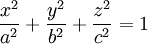$\frac{x^2}{a^2} + \frac{y^2}{b^2} + \frac{z^2}{c^2} = 1$

where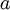$a$,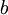$b$ and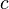$c$ define the lengths of the axis.

## Overlap algorithm

The most widely used overlap algorithm is that of Perram and Wertheim .

## Geometric properties

The mean radius of curvature is given by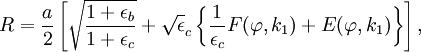$R= \frac{a}{2} \left[ \sqrt{\frac{1+\epsilon_b}{1+\epsilon_c}} + \sqrt \epsilon_c \left\{ \frac{1}{\epsilon_c} F(\varphi , k_1) + E(\varphi,k_1) \right\}\right],$

and the surface area is given by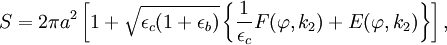$S= 2 \pi a^2 \left[ 1+ \sqrt {\epsilon_c(1+\epsilon_b)} \left\{ \frac{1}{\epsilon_c} F(\varphi , k_2) + E(\varphi,k_2)\right\} \right],$

where$F(\varphi,k)$ is an elliptic integral of the first kind and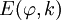$E(\varphi,k)$ is an elliptic integral of the second kind, with the amplitude being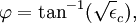$\varphi = \tan^{-1} (\sqrt \epsilon_c),$

and the moduli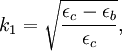$k_1= \sqrt{\frac{\epsilon_c-\epsilon_b}{\epsilon_c}},$

and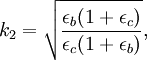$k_2= \sqrt{\frac{\epsilon_b (1+\epsilon_c)}{\epsilon_c(1+\epsilon_b)}},$

where the anisotropy parameters,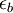$\epsilon_b$ and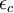$\epsilon_c$, are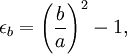$\epsilon_b = \left( \frac{b}{a} \right)^2 -1,$

and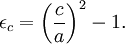$\epsilon_c = \left( \frac{c}{a} \right)^2 -1.$

The volume of the ellipsoid is given by the well known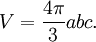$V = \frac{4 \pi}{3}abc.$

## Maximum packing fraction

Using event-driven molecular dynamics, it has been found that the maximally random jammed (MRJ) packing fraction for hard ellipsoids is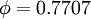$\phi=0.7707$ for models whose maximal aspect ratio is greater than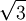$\sqrt{3}$  

## Equation of state

Main article: Hard ellipsoid equation of state

## Virial coefficients

Main article: Hard ellipsoids: virial coefficients

## Phase diagram

One of the first phase diagrams of the hard ellipsoid model was that of Frenkel and Mulder (Figure 6 in ). Camp and Allen later studied biaxial ellipsoids . It has recently been shown   that the SM2 structure is more stable than the face centered cubic structure for aspect ratios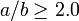$a/b \ge 2.0$ and densities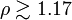$\rho \gtrsim 1.17$.

## Hard ellipse model

Main article: Hard ellipse model (2-dimensional ellipsoids)